neutrino

(redirected from Tauon neutrino)
Also found in: Dictionary, Thesaurus, Medical.

neutrino

(no͞otrē`nō) [Ital.,=little neutral (particle)], elementary particleelementary particles,
the most basic physical constituents of the universe. Basic Constituents of Matter

Molecules are built up from the atom, which is the basic unit of any chemical element. The atom in turn is made from the proton, neutron, and electron.
with no electric charge and a very small mass emitted during the decay of certain other particles. The neutrino was first postulated in 1930 by Wolfgang PauliPauli, Wolfgang
, 1900–1958, Austro-American physicist, b. Vienna. He studied first with A. Sommerfeld at Munich and then with Niels Bohr at Copenhagen. After lecturing (1923–28) at the Univ.
in order to maintain the law of conservation of energy during beta decay (see conservation lawsconservation laws,
in physics, basic laws that together determine which processes can or cannot occur in nature; each law maintains that the total value of the quantity governed by that law, e.g., mass or energy, remains unchanged during physical processes.
spontaneous disintegration or decay of the nucleus of an atom by emission of particles, usually accompanied by electromagnetic radiation. The energy produced by radioactivity has important military and industrial applications.
). When a radioactive nucleus emits a beta particle (electron), the electron may have any energy from zero up to a certain maximum. Pauli suggested that when the electron has less than the maximum possible value, the remaining energy is carried away by an undetected particle, the neutrino. Its charge must be zero because a charged particle would easily be detected. Moreover, if it were charged, the law of conservation of charge would be violated during beta decay. The neutrino was named by Enrico FermiFermi, Enrico
, 1901–54, American physicist, b. Italy. He studied at Pisa, Göttingen, and Leiden, and taught physics at the universities of Florence and Rome. He contributed to the early theory of beta decay and the neutrino and to quantum statistics.
. Further studies showed that the neutrino was also necessary to maintain the conservation laws of momentum and spin. Like the electron, the neutrino is a leptonlepton
[Gr.,=light (i.e., lightweight)], class of elementary particles that includes the electron and its antiparticle, the muon and its antiparticle, the tau and its antiparticle, and the neutrino and antineutrino associated with each of these particles.
; it participates only in the weak decay of nuclear particles and has no role in the strong force binding nuclei together. Neutrinos are also emitted when a pionpion
or pi meson,
lightest of the meson family of elementary particles. The existence of the pion was predicted in 1935 by Hideki Yukawa, who theorized that it was responsible for the force of the strong interactions holding the atomic nucleus together.
decays into a muonmuon
, elementary particle heavier than an electron but lighter than other particles having nonzero rest mass. The name muon is derived from mu meson, the former name of the particle. The muon was first observed in cosmic rays by Carl D.
and in the decays of a number of other elementary particles. Neutrinos are stable and can be absorbed only by the same weak interactionsweak interactions,
actions between elementary particles mediated, or carried, by W and Z particles and that are responsible for nuclear decay. Weak interactions are one of four fundamental interactions in nature, the others being gravitation, electromagnetism, and the strong interactions.
through which they are created; an energetic neutrino can induce the reverse of the decay that produced it.

The neutrino was not detected directly until 1956, when American physicists Frederick Reines and Clyde L. Cowan recognized them by their impact with subnuclear particles in mineral water. In 1962 it was found that the neutrino associated with the muon (the muon neutrino) is distinct from that associated with the electron (the electron neutrino). A third type, the tau neutrino, associated with the tau particle, was identiified in the mid-1970s but not detected until 2000. Each type of neutrino has its own antiparticle.

According to the so-called oscillation theory, neutrinos can change from one type to another as they travel through space; in order to make these transformations, neutrinos have to have a tiny amount of mass and not be massless, as was originally theorized. Beginning in the late 1960s a number of experiments designed to detect neutrinos failed to produce the expected results when fewer than expected neutrinos were detected, a result that could be explained by the conversion of the type (or flavor) of neutrino the experiments were trying to detect into another type, a process known as flavor oscillation. In 1995 and again in 1996 a team at the Los Alamos National Laboratory claimed to have detected the oscillation of muon antineutrinos into electron antineutrinos, and in 1998 the participants in the Super-Kamiokande experiment in Japan, which examined neutrinos produced by the interaction of cosmic rays with the upper atmosphere, announced that they had discovered evidence that neutrinos oscillate and must have mass. In 2001 researchers at the Sudbury Neutron Observatory in Ontario, Canada, found evidence that the electron neutrinos produced by fusion reactions within the sun can change into tau and muon neutrinos as they travel to the earth. Additional work by Fermilab in Illinois and Minnesota confirmed (2006) that neutrinos have mass. This is significant because of its implications for the composition and evolution of the universe, including the rate of the universe's expansion. Neutrinos would exert gravitational effects and thus could account for some of the dark matterdark matter,
material that is believed to make up nearly 27% of the mass of the universe but is not readily visible because it neither emits nor reflects electromagnetic radiation, such as light or radio signals.
in the universe.

study of stars by means of their emission of neutrinos, fundamental particles that result from nuclear reactions and are emitted by stars along with light. Approximately 100 billion neutrinos have raced through your body since you began reading this article.
.

Neutrino

An elusive elementary particle that interacts with matter principally through the weak nuclear force. Neutrinos are electrically neutral spin-½ fermions with left-handed helicity. Many weak interaction processes (interactions that involve the weak force), such as radioactive nuclear beta decay and thermonuclear fusion, involve neutrinos. Present experimental knowledge is consistent with neutrinos being point particles that have no internal constituents. Neutrinos are classified as neutral leptons, where leptons are defined as elementary particles that interact with the electroweak (electromagnetic and weak nuclear) and gravitational forces but not with the strong nuclear force. See Elementary particle, Fundamental interactions, Helicity (quantum mechanics), Lepton, Spin (quantum mechanics), Weak nuclear interactions

Because the role of gravitational forces is negligible in nuclear and particle interactions and because neutrinos have zero electric charge, neutrinos have the unique property that they interact almost completely via the weak nuclear force. Consequently, neutrinos can be used as sensitive probes of the weak force. As such, neutrino beams at particle accelerators have been employed to study charge-changing (charged current) and charge-preserving (neutral current) weak interactions. However, the extreme weakness (compared to the electromagnetic and strong forces) and short range (of the order of 10-18 m) of the weak interaction have made determination of many neutrino properties extremely difficult.

Currently, three distinct flavors (or types) of neutrinos are known to exist: the electron neutrino (&ngr;e), the muon neutrino (&ngr;μ), and the tau neutrino (&ngr;&tgr;). Each neutrino flavor is associated with a corresponding charged lepton, the electron (e), muon (μ), and tau (&tgr;) particle. The electron, muon, and tau neutrinos (or their antiparticles) have been observed in experiments. Based on present measurements, the lepton flavor families, which comprise the charged and neutral leptons and their antiparticles (e-, &ngr;e, e+, e; μ-, &ngr;μ, μ+, μ; &tgr;-, &ngr;&tgr;, &tgr;+, &tgr;), obey laws of conservation of lepton number. These empirical laws state that the number of leptons minus antileptons does not change, both within a flavor family and overall. See Electron, Symmetry laws (physics)

The existence of neutrino oscillations (a phenomenon whereby neutrinos change their flavors during the flight from a neutrino source to a detector), seen clearly in observations of atmospheric neutrinos, shows that neutrinos have tiny finite masses which are many orders of magnitude smaller than the masses of their charged lepton counterparts, and also shows that the physical neutrinos do not have pure flavors (quantum-mechanical states) but contain mixtures of two or more neutrino states. This mixing indicates that the empirical laws of lepton number conservation are not exact and that they are violated in some physical processes. It is not known whether neutrinos have magnetic or electric dipole moments.

McGraw-Hill Concise Encyclopedia of Physics. © 2002 by The McGraw-Hill Companies, Inc.

neutrino

(new-tree -noh) Symbol: ν. An elementary particle with zero charge and spin ½. There are three types of neutrino, νe, νμ, and ντ, which are associated with the electron, muon, and massive tau lepton respectively; the latter three particles together with the three neutrinos make up the family of leptons. Neutrinos are thought to have either zero mass or a very small mass. The antiparticle of the neutrino is the antineutrino (¯ν). Neutrinos have only a weak interaction with matter. See also neutrino astronomy.
Collins Dictionary of Astronomy © Market House Books Ltd, 2006
The following article is from The Great Soviet Encyclopedia (1979). It might be outdated or ideologically biased.

Neutrino

an electrically neutral elementary particle with a rest mass much less than the mass of the electron (possibly equal to zero), a spin of 1/2 (in units of Planck’s constant ℏ), and a vanishingly small (apparently zero) magnetic moment. The neutrino belongs to the lepton family, and, by its statistical properties, to the fermion class.

The term “neutrino” is applied to two different elementary particles—the electronic neutrino (ve) and the muonic neutrino (vµ). A neutrino that in interactions with other particles is paired with an electron e- or with a positron e+ is called an electronic neutrino, while a neutrino that in interactions is paired with a muon - or μ+) is called a muonic neutrino. Both types of neutrinos have corresponding antiparticles: the electronic antineutrino (ve) and the muonic antineutrino (v̄μ). The additive lepton quantum numbers (lepton charges) Le and Lµ, which are conserved, enable us to distinguish between electronic neutrinos and muonic neutrinos. Here, it is assumed that Le = +1 and Lμ = 0 for ve, Le = —1 and Lµ = 0 for v̄e, Le = 0 and L µ= + 1 for vμ, and Le = 0 and Lµ = — 1 for v̄μ. In contrast to other particles, neutrinos have the surprising property of a strictly defined value of helicity λ—the component of the spin in the direction of the linear momentum. Neutrinos have left-handed helicity (λ=– 1/2); that is, the spin is directed opposite to the motion of the particle. Antineutrinos, however, have right-handed helicity (λ = + 1/2); that is, the spin is directed along the motion.

Neutrinos are emitted during beta decay of atomic nuclei, during K capture, during the capture of µ- by nuclei, and during decays of unstable elementary particles—chiefly pions, or pi-mesons (π+ and π-); kaons, or K-mesons; and muons, or mu-mesons. Thermonuclear reactions in stars are also sources of neutrinos.

Neutrinos participate in weak interactions and in gravitational interactions but not in electromagnetic and strong interactions. This quality of the neutrino is responsible for its exceedingly high penetrating power, which allows it to pass freely through the earth and the sun.

Pauli’s hypothesis. The discovery of the neutrino is among the most brilliant yet most difficult accomplishments of 20th-century physics. Before becoming an accepted member of the family of elementary particles, the neutrino long remained a hypothetical particle.

The neutrino first appeared in experimental physics in 1914, when the British physicist J. Chadwick observed that the electrons emitted during beta decay of atomic nuclei have a continuous energy spectrum, in contrast to the alpha particles and gamma quanta that are emitted during other types of radioactive transmutations. This phenomenon was in obvious contradiction to the quantum theory, since the quantum theory required that a discrete amount of energy be released in quantum transitions between stationary states of nuclei (Bohr postulate). Since this requirement was satisfied in the emission of alpha particles and gamma quanta, the validity of the law of conservation of energy during beta decay began to be questioned.

In 1930, the Swiss physicist W. Pauli reported, in a letter to the participants of a seminar at Tübingen, his “desperate attempt” to “save” the law of conservation of energy. Pauli advanced the hypothesis that there exists a new, electrically neutral, strongly penetrating particle with spin 1/2 and mass < 0.01 of the mass of a proton; this new particle is emitted together with an electron during beta decay. This situation leads to a disruption of the homogeneity of the energy spectrum of the beta electrons as a result of the division of the discrete amount of energy (corresponding to the transition of the nucleus from one state to another) between the two particles. After the discovery, in 1932, of a heavy neutral particle—called the neutron—the Italian physicist E. Fermi proposed the name “neutrino” for Pauli’s particle. In 1933, Pauli stated the basic properties of the neutrino in their present-day form. As was evident later, this hypothesis “saved” not only the law of conservation of energy but also the laws of conservation of momentum and conservation of angular momentum, as well as the fundamental principles of particle statistics in quantum mechanics.

Fermi’s theory of beta decay. Pauli’s hypothesis fitted in a natural way into the theory of beta decay that was proposed by Fermi in 1934. This theory made it possible to describe the phenomena of electron (β-) decay, positron (β+) decay, and K capture. The existence of two different neutrinos now became a theoretical possibility: (1) the antineutrino, which is created along with an electron, and (2) the neutrino, which is created along with a positron.

In Fermi’s theory, β- decay (or β+ -decay) is the conversion of a neutron n (or a proton p) inside a nucleus into a proton (or a neutron):

(1) n →p + e- + v̄e

(2) p →n + e+ + ve

The shape of the spectrum of the beta electrons was calculated by means of Fermi’s theory and proved to be very sensitive to the mass mv of the neutrino near the upper limit of the energy of the beta electrons. A comparison of the theoretical and the experimental shape of the spectrum showed that the mass of the neutrino is much less than the electron mass (and may, possibly, be equal to zero). Fermi’s theory explained all the basic features of beta decay, and the success of the theory led physicists to recognize the neutrino. However, doubts as to the existence of this particle remained.

Neutrino-detection experiments. Two possible methods of experimentally detecting neutrinos are known. The first method is the observation of inverse beta decay; this method was first considered by H. Bethe and R. Peierls in 1934. Inverse beta decay is the name given to the reactions

(1’) n + ve → p + e-

(2’) p + v̄e → n + e+

whose existence follows from Fermi’s theory; these reactions occur both in free nucleons and in nucleons bound in nuclei. An estimate of the probability, or cross section, of the neutrino absorption gave an astonishing result: in a solid, a neutrino having an energy characteristic of beta decay should traverse a distance of the order of hundreds of light-years before being captured by a nucleus. In the 1930’s and 1940’s, the detection of such a particle seemed to be an impossibility.

The second method is the observation of the recoil of a nucleus at the instant of neutrino emission; this method was first considered by the Soviet physicist A. I. Leipunskii. In 1938, A. I. Alikhanov and A. I. Alikhan’ian proposed, for this purpose, the use of the K-capture reaction in Be. The 7Be nucleus captures an electron from the K shell of the atom and emits a neutrino, the 7Be nucleus being transmuted into a 7Li nucleus: 7Be (e-, ve)7Li. In this case, if the neutrino is a real particle, the 7Li nucleus gains momentum equal in magnitude and opposite in sign to the momentum of the neutrino. The first successful experiment using this reaction was carried out in 1942 by the American physicist J. Allen. The recoil energy of the 7Li ions proved to be in agreement with the theoretical value (if the neutrino was assumed to have zero mass). Subsequent experiments confirmed this result with greater accuracy. Thus, the existence of neutrinos had become an experimental fact. A new particle all of whose properties were determined from indirect experiments had appeared in physics.

The detection of a free neutrino in the process of inverse beta decay became possible after the development of powerful nuclear reactors and large hydrogen-containing scintillation counters. In a reactor, antineutrinos with energies up to 10 megaelectron volts (MeV) are emitted as a result of the β- -decay of the fission fragments of uranium; there is an average of six particles per fission. The antineutrino flux from a powerful reactor measures about 1013 particles/(cm2·sec) near the reactor.

An experiment designed for the direct detection of ve was first carried out in the USA in 1953 by F. Reines and C. Cowan at the Hanford reactor. Reaction (2’) in the hydrogen contained in the scintillator liquid was detected by adding a cadmium salt, which strongly absorbs neutrons. The characteristic chain of events initiated by the antineutrino was separated from the background by means of a delayed-coincidence technique: a positron created in reaction (2’), upon annihilation with an electron, emits two gamma quanta, which cause the first scintillation; after 5–10 μsec, there is a second scintillation, which is caused by the gamma quanta that are emitted by a cadmium nucleus as a result of the capture of the neutron that is created in reaction (2’) and decelerated in the hydrogen-containing liquid. The experiment was repeated under better conditions in the period from 1956 to 1959. A diagram of the 1958 experiment is shown in Figure 1. A cross section of σ = (11 ± 2.6) × 10-44 cm2 was obtained. The theoretical value of the cross section averaged over the antineutrino spectrum is equal to (10–14) × 10-44 cm2, if we assume a two-component neutrino (see below). These experiments definitely confirmed the existence of the free neutrino.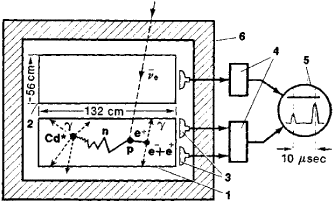Figure 1. Diagram of 1958 experiment of Reines and Cowan carried out with a reactor at the Savannah River Plant: (1) liquid-scintillator detector (1,400 liters) for detecting antineutrinos; (2) scintillation counter for recording the cosmic-ray background, connected for anticoincidence with detector (1); (3) two groups of photomultipliers connected for coincidence; (4) electronic equipment; (5) double-beam oscillograph; (6) lead and paraffin shields against reactor radiation

Neutrino and antineutrino. The concept of the neutrino and the antineutrino arose in a purely theoretical fashion. However, proof that these particles are actually different cannot be obtained within the framework of the theory itself. Since the neutrino does not have an electric charge, we cannot exclude the possibility that the neutrino is identical to the antineutrino in its properties, that is, it is really a neutral particle. Such a neutrino was first considered by the Italian physicist E. Majorana and is therefore called the Majorana neutrino. In 1946, B. M. Pontecorvo proposed the use of the transmutation of 37Cl into 37Ar for the experimental solution of this problem. The reaction

(3) 37Cl + ve37Ar + e-

follows from the existence of the decay 37Ar (e-, ve)37Cl. If ve and v̀e are not identical, then the reaction

(4) 37Cl + v̀e37Ar + e-

which is similar to reaction (3), should not be observed when 37Cl is irradiated by an antineutrino beam from a reactor. The reaction (4) was not observed in an experiment conducted by the American scientist R. Davis in 1955–56 using carbon tetrachloride. This result proved that ve and v̀e are not identical; the result is thus the basis for introducing the lepton number Le, which is conserved.

Electronic neutrinos and muonic neutrinos. It was established after the discovery of muons, pions, and kaons that the decay of these particles is also accompanied by the emission of neutrinos:

μ± → e± + v + v̀, μ± + v, K± → μ± + v

In 1957, M. A. Markov, J. Schwinger, and K. Nishijima advanced the hypothesis that the neutrino created along with a muon (vμ) differs from the neutrino created along with an electron (ve). The possibility of verifying these associative properties of neutrinos by using high-energy accelerators was considered in the USSR by Markov and Pontecorvo. Successful experiments were carried out in 1962 at the Brookhaven accelerator in the USA and in 1964 at the European Center (Organization) for Nuclear Research (CERN). Results showed that only the reaction vμ + n → + μ- takes place under the action of neutrinos from the decays

(5) π+ → μ + vμ, K+ → μ+ + vμ

The reaction vμ + n → p + e- was not observed; this means that the neutrinos from reactions (5) do not create electrons. Thus, the existence of two different neutrinos—νμ and ve—had been proved.

From 1964 to 1967, it was established in similar experiments that when vμ collide with nuclei, μ- are created but μ+ are not, that is, the muonic neutrino vμ and the muonic antineutrino v̀μ are not identical, and an additional lepton number, Lμ, which is conserved, must be introduced.

Helicity and lepton numbers of neutrino. Before the discovery of the nonconservation of parity in beta decay, it was assumed that the neutrino is described by a wave function that is a solution of the Dirac equation and has four states that correspond to the four linearly independent solutions: two with the spin component on the momentum (helicity) λ = –1/2—the left-handed neutrino t and the left-handed antineutrino t and two with λ = +1/2—the right-handed neutrino v̄r and the right-handed antineutrino v̄r. The theory of the neutrino that assumes the existence of four states is called the four-component theory, while the theory that assumes two states is called the two-component theory. The Majorana neutrino is an example of a two-component neutrino.

The discovery of the nonconservation of parity in 1956 opened up a new theoretical possibility for describing neutrinos. In 1957, L. D. Landau and, independently, the Pakistani physicist A. Salam, as well as T. D. Lee and C. N. Yang, constructed a two-component theory of the helical neutrino in which the neutrino has only two states: either v t and v̀r or vr and v̀t that is, the neutrino and antineutrino have helicities of opposite value. For a helical, two-component neutrino, the operation of space inversion P (the operation of the conversion from a right-handed coordinate system to a left-handed system) and the operation of charge conjugation C (the conversion from particle to antiparticle) do not have physical meaning when taken separately, since they convert a real neutrino into a nonphysical state of incorrect helicity. Only the product of these operations, called combined parity (CP), which transforms a real neutrino vt (or vr) into a real antineutrino v̀r (or t) of opposite helicity, has physical meaning.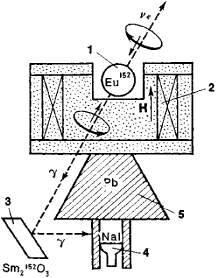Figure 2. Diagram of experiment to measure helicity of neutrinos: (1) radioactive preparation 152Eum(Jπ: : O- (where J is spin and π is parity of nucleus), (2) magnetic analyzer (magnetized iron), (3) 152Sm (O+) nuclei, (4) Nal scintillation counter, (5) lead shield

The helicity of the electronic neutrino emitted in the process 152Eum(e-, ve) 152Sm* was measured directly at Brookhaven in 1958 by the American physicists M. Goldhaber, L. Grodzins, and A. Sunyar. A diagram of the experiment is shown in Figure 2. The radioactive preparation 152Eum(Jπ = 0-), denoted by (1) in the figure, emits a neutrino during K capture; J is the spin and π is the parity of the nucleus. The resulting excited nucleus 152Sm* (1-) emits a gamma quantum [thus being transmuted into a 152Sm (0 +) nucleus], which, on passing through the magnetic analyzer (2) (magnetized iron), undergoes resonance scattering by 152Sm (0 +) nuclei (3). The resonance condition is satisfied only if the Sm nucleus has a small recoil momentum after it emits a gamma quantum, that is, if the neutrino and gamma quantum are emitted in opposite directions. In this case, the gamma quantum and neutrino must have helicity of the same sign. The NaI scintillation counter (4) counts the number of scattered gamma quanta N+ and N- when the magnetic field is along and opposite to the motion of the neutrinos. The theoretical value of (N – N+)/2(N + N+) is +0.025 for left-handed helicity and –0.025 for right-handed helicity; the experimental value is +0.017 ± 0.003, which is consistent with all of the neutrinos having left-handed helicity if all possible effects of depolarization of the gamma quanta are taken into account. [The lead shield (5) protects the counter (4) from direct incidence of gamma quanta.] The results of the experiment showed that, with a probability close to 100 percent, ve has left-handed helicity. Measurements of the helicity of muonic neutrinos in the decay π+ → μ+ + vμ showed that vμ is also left-handed. It was also established that v̀e and v̀μ have right-handed helicity (Figure 3).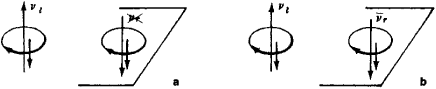Figure 3. (a) Upon mirror reflection (space inversion), the left-handed neutrino vt would be converted into the nonexistent state of a right-handed neutrino vr; (b) a real state is obtained if the reflection of the neutrino is accompanied by a simultaneous conversion of the neutrino from a particle to an antiparticle, with v1 becoming a right-handed antineutrino v̀r in the process

These experiments, however, are insufficient to confirm the theory of the two-component neutrino. The experiments of Reines and Cowan, which were designed to measure the antineutrino capture cross section (see above) are proof of the two-component character of the neutrino: the cross section calculated according to the two-component theory proved to be twice as great as the cross section calculated according to the four-component theory. Although none of the experiments conducted with neutrinos exclude the Majorana version of the two-component neutrino, the theory of the helical two-component neutrino is preferable, since it permits introduction of the lepton numbers Le and Lμ, which are used to obtain all necessary prohibitions in processes involving leptons, such as µ± ↛ e± + γ, e- + p ↛ n + π- + μ+, and K- ↛ π+ + e- + μ-. The helical two-component theory is logically more orderly and “economical,” since the zero mass and zero magnetic moment of the neutrino stem naturally from this theory.

In addition to Le and Lμ, there are lepton numbers introduced by other methods.

Mass and magnetic moment of neutrino. It is experimentally impossible to exclude the possibility that the neutrino has a very small mass. The best estimate of the upper limit of the mass of the electronic neutrino has been obtained by an analysis of the shape of the β-electron spectrum of tritium and is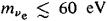This is less than the mass of the electron by a factor of almost 104 (me ≈ 510 keV). For the muonic neutrino, the experimental limit is much higher: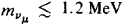If the mass of the neutrino is not strictly equal to zero, the neutrino can have a magnetic moment and consequently can take part in electromagnetic interactions, such as the reactions

ve + e- → ve + e-, νμ + ρ → ρ + π0 + νμ

Experiments aimed at detecting these reactions have given the following upper limits on the value of the magnetic moment: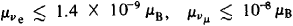where μB is the Bohr magneton. If

mve = mv μ = 0

then

Mve = μ = 0

Neutrino oscillations. In 1958, Pontecorvo advanced the hypothesis that if the mass of the neutrino is not strictly equal to zero and if lepton charges are not strictly conserved, then neutrino oscillations are possible. Such oscillations are the conversion of one type of neutrino into another (in a manner similar to the K0 ⇄ K0 oscillations of kaons as a result of the nonconservation of strangeness in weak interactions), such as ve ⇄ v̀e and ve ⇄ νμ. The problem of oscillations can be solved only by experimental means.

As was stated above, neutrinos interact with other particles by means of the weak interaction. The present theory of universal weak interaction (the generalized Fermi theory), which was developed by the American scientists M. Gell-Mann, R. Feynman, R. Marshak, and E. Sudarshan, describes all experimentally observed processes involving neutrinos; this theory also predicts processes that have not yet been observed, such as the elastic scattering of a neutrino by an electron or by a muon: ve + e → ve + e or νμ + μ → νμ + μ. Experiments on the scattering of neutrinos by electrons have attained a sensitivity nearly sufficient for detecting these processes, but it has not yet been possible to isolate these processes from the background noise.

The interaction of neutrinos at high energies is of particular interest. According to the present theory of the weak interaction, the cross section of the scattering of neutrinos by other leptons, such as the νμ + e- → ve + μ- reaction, should increase with increasing energy as the square of the energy of the colliding particles in the center-of-mass system (or linearly with the energy in the laboratory system). In the local Fermi theory, however, the interaction cross section cannot increase without bound, since at energies of the order of 300 GeV the cross section in the center-of-mass system reaches its natural limit, which is defined by the unitarity condition (the condition that the total probability of all possible processes upon collision of these particles be equal to unity). It may be expected that at these energies, if the present theory proves valid, the weak interaction will become “strong” in the sense that the cross sections of processes of multiple production of leptons will become comparable to the cross section of two-particle processes.

At present, only processes involving the interactions of neutrinos with strongly interacting particles (hadrons) have been investigated experimentally. Quasi-elastic processes of the type νeμ) + n → p + e--) and inelastic processes such as νeμ) + n → n (p) + e--) + Nπ + N’K + . . ., where N and N’ are integers, have been observed. For quasi-elastic processes, the behavior of the cross section with increasing energy can be theoretically predicted. According to the hypothesis of the Soviet scientists S. S. Gershtein and Ia. B. Zel’dovich, the nucleon is the carrier of a “weak charge,” which is conserved and which is similar to electric charge. If this is the case, then the “weak charge” (like the electric charge) should be “spread” throughout the volume of the nucleon, and the nucleon when it interacts with a neutrino should behave like an extended particle. While the cross section for quasi-elastic scattering of neutrinos by a point nucleon increases linearly with increasing energy (in the laboratory system), calculations show that for scattering by an extended nucleon the cross section reaches a constant value at a neutrino energy Ev = 1–2 GeV. Experiments have confirmed this hypothesis for Ev = 1–5 GeV.

The situation is more complex for inelastic processes. Markov advanced the hypothesis that the total cross section for the interaction of a neutrino with a nucleon should increase linearly with increasing energy (in the laboratory system) because of the unlimited increase in the number of possible channels, despite a cutoff of the cross section in each individual channel of the reaction. This was proved, within the framework of certain assumptions, by the American scientists S. Adler and J. Bjorken. As Feynman has shown, such an energy dependence of the cross section is possible if the nucleon is a cloud of point particles (“partons”).

Measurements made at CERN are consistent with a linear increase in the total cross section in the region Ev = 1–10 GeV: σν = (0.69 ± 0.05) × 10-38Ev cm2 (in this equation, the energy Ev is expressed in gigaelectron volts). Data have also been obtained in experiments with neutrinos in cosmic rays at energies of 10–100 GeV: σv = (0.55 ± 0.15) × 10-38Ev cm2. Preliminary results obtained at the National Accelerator Laboratory of the USA (Batavia, III.) are not at variance with a linear increase in the cross section up to Ev≃ 40 GeV. Thus, all the data are in accord with a linear increase in the total cross section for the interaction of a neutrino with a nucleon for Ev ≥ 100 GeV. It has been hypothesized that the cross section may increase linearly with energy up to the geometrical dimensions of the nucleon (≃10-26 cm2).

There is a theory in which the weak interaction is accomplished through the exchange of an intermediate boson. In this theory, which differs from Fermi’s theory, the cross section for the interaction of a neutrino both with leptons and with hadrons should have a cutoff at high energies. The cutoff energy is determined by the mass of the intermediate boson.

In 1973 about 100 cases of the interaction of vμ and v̄μ with nuclei, resulting in the creation of hadrons without muons, were observed for the first time in a bubble chamber at CERN; several cases of the scattering of v̄μ by an electron were also observed there in 1974. This apparently indicates the existence of a new type of interaction of neutrinos with hadrons and leptons through so-called neutral currents. The existence of such interactions follows, in particular, from the unified theory of weak and electromagnetic interactions.

In all the experiments discussed above, the neutrino assumes the role of a tool for investigating the structure of elementary particles.

Natural radioactivity. Any cosmic body, including the earth, contains a large number of radioactive elements and is a source of neutrinos. In principle, it is possible to detect antineutrinos from the earth, but methods of detection have not yet been developed.

Collision of cosmic-ray protons with a gas and with residual photons. The collision of cosmic-ray protons with a gas and with residual photons may lead to the creation of charged pions, whose decay is accompanied by the emission of neutrinos (or antineutrinos). Neutrinos with energies up to Ev = 1020 eV can be produced by this mechanism. The galactic nucleus and disk, where most of the interstellar gas is concentrated, and the earth’s atmosphere are sources of such neutrinos. Neutrinos from the collision of superhigh-energy protons with residual photons are emitted over the whole universe. According to one hypothesis, superhigh-energy neutrinos are the cause of high-power, extensive air showers.

The earth’s atmosphere is thus far the only natural source from which it has been possible to detect neutrinos. Neutrinos are created in the upper layers of the atmosphere, where the greatest number of pions and kaons are generated. The idea of conducting experiments with cosmic-ray neutrinos was first advanced by Markov in 1960. He proposed the detection deep underground of muons with an energy of 10–100 GeV from the reaction

(6) vμ + n → p + μ-

By detecting muons from the earth’s lower hemisphere at large zenith angles, it is possible to eliminate atmospheric muons from the background and obtain pure neutrino events (6). Results were first obtained in India and South Africa in 1965 by special neutrino telescopes. One of the experiments is shown in Figure 4. The experiment was carried out in a mine in southern India at a depth of about 2,300 m. The plastic scintillation elements (1) all have an area of 1 m2 each and are viewed with two photomultipliers (2); quadruple coincidences between a pair of photomultipliers on one side and any pair on the other side are recorded; several layers of neon tubes (3) are mounted between the scintillators for photographing the tracks of charged particles created by neutrinos; the lead absorbers (4) are each 2.5 cm thick. By 1973, more than 100 neutrino events had been detected throughout the world.

Thermonuclear-fusion reactions. Thermonuclear-fusion reactions of chemical elements are the primary mechanism by which neutrinos are generated in the interior of the sun and most stars (during the period of the “nuclear” evolution of the sun and these stars).

Ultrahot plasma. Ultrahot plasma is a source of neutrinos in stars in the final stages of their evolution and also in the primordial fireball model of the origin of the universe during the first fractions of a second of its emergence. Two types of neutrino generation are possible. The first is associated with reactions of the interconversion of nucleons p ⇄; n (the URCA process) and may take place both in bound nucleons of nuclei at temperatures of T ≃ 109 °K and in free nucleons at T < 1010 °K. The second method, a purely leptonic method, is associated with reactions of the type μ-e- + ̄e +e + vμ and also with such reactions as γ +ee + vee (photoproduction of neutrinos) and e+ + e- → ve + ̄e (neutrino annihilation of electron-positron pairs), which occur if the hypothesized scattering ve + eve + e (which is predicted by Fermi’s theory) exists. The existence of ve + eve + e scattering has not yet been proved by laboratory methods (with neutrinos from reactors and accelerators); it is believed that astrophysical data support the existence of this process.

RESIDUAL NEUTRINOS. According to the primordial fireball model, neutrinos emitted at the instant of formation of the universe undergo a strong red shift during cosmological expansion of the universe. Such residual neutrinos fill all space. In the most realistic version of the primordial fireball model, the number of muonic neutrinos and electronic neutrinos is equal to the number of muonic antineutrinos and electronic antineutrinos (≃200 particles/cm3), and the average energy of the neutrinos is (2–3) X 10-4 eV, which corresponds to a neutrino gas at a temperature of 2–3°K. In order to understand the mechanism of the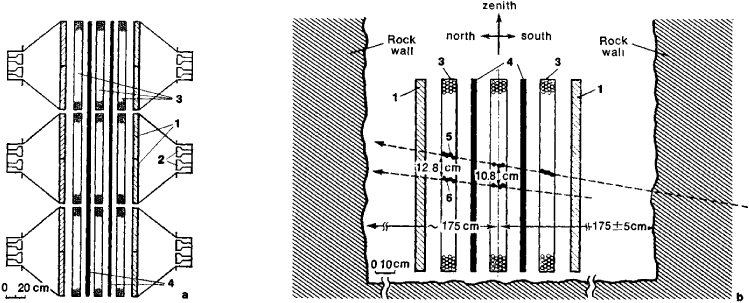Figure 4.(a) Diagram of neutrino telescope set up in a mine: (1) plastic scintillation elements, (2) photomultipliers, (3) neon tubes, (4) lead absorbers; (b) the case of inelastic interaction of a neutrino from the earth’s lower hemisphere: (5) and (6) tracks apparently left by a muon and a pion produced within the rock upon collision of a v μ with a nucleon

evolution of the universe, it is very important to establish experimentally the existence of residual neutrinos and to measure the temperature of the neutrino gas.

The best estimate for the mass of the muonic neutrino can be obtained within the framework of the primordial fireball model. According to cosmological data, the density of matter in the expanding universe cannot exceed 10-28 g/cm3. Therefore it follows that the maximum possible mass of the muonic neutrino is ≃ 300 eV, that is, much less than the upper limit established by laboratory methods.

Neutronization of matter. The neutronization of matter, that is, the conversion of protons into neutrons according to the scheme p + e- → n + ve, can serve as a powerful source of neutrinos when, for various reasons, a star loses its gravitational stability and collapses, becoming a neutron star. In the process, an enormous number of neutrinos, equal in order of magnitude to the number of protons in the star (≃1057), are emitted within hundredths of a second. If a hot star collapses, neutronization takes place in conjunction with processes that are characteristic of a hot plasma. Such a situation is possible during outbursts of supernovae and during gravitational collapse.

See for a discussion of the possibility of detecting neutrinos from the sun and other stars.

The development of neutrino science in the past quarter century has convincingly proved that the neutrino has progressed in status from a hypothetical particle to a powerful tool for investigating the microcosm and macrocosm.

REFERENCES

Allen, J. S. Neitrino. Moscow, 1960. (Translated from English.)
Alikhanov, A. I. Slabye vzaimodeistviia: Noveishie issledovaniia β-ras-pada. Moscow, 1960.
Teoreticheskaia fizika 20 veka. Moscow, 1962.
Okun’, L. B. Slaboe vzaimodeistvie elementarnykh chastits. Moscow, 1963.
Pontecorvo, B. M. “Neitrino i ego rol’ v astrofizike.” Uspekhi fizicheskikh nauk,1963, vol. 79, issue 1, p. 3.
Markov, M. A. Neitrino. Moscow, 1964.
Zheleznykh, I. M. “Podzemnye neitrinnye eksperimenty.” Uspekhi fizicheskikh nauk,1966, vol. 89, issue 3, p. 513.
Lee, T., and C. S. Wu. Slabye vzaimodeistviia. Moscow, 1968. (Translated from English.)
Bugaev, E. V., Iu. D. Kotov, and I. L. Rozental’. Kosmicheskie miuony i neitrino. Moscow, 1970.
Berezinskii, V. S. Neitrino. Moscow, 1973.

G. T. ZATSEPIN and IU. S. KOPYSOV

neutrino

[nü′trē·nō]
(physics)
A neutral particle having zero rest mass and spin ½ (h /2π), where h is Planck's constant; experimentally, there are two such particles known as the e neutrino (νe ) and the μ neutrino (νμ).
McGraw-Hill Dictionary of Scientific & Technical Terms, 6E, Copyright © 2003 by The McGraw-Hill Companies, Inc.

neutrino

Physics a stable leptonic neutral elementary particle with very small or possibly zero rest mass and spin ½ that travels at the speed of light. Three types exist, associated with the electron, the muon, and the tau particle
Collins Discovery Encyclopedia, 1st edition © HarperCollins Publishers 2005
Site: Follow: Share:
Open / Close